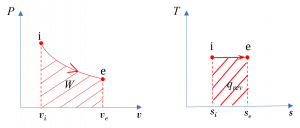6. Entropy and the Second Law of Thermodynamics

# 6.9 The second law of thermodynamics for open systems

Entropy can be transferred to a system via two mechanisms: (1) heat transfer and (2) mass transfer. For open systems, the second law of thermodynamics is often written in the rate form; therefore, we are interested in the time rate of entropy transfer due to heat transfer and mass transfer.

$\dot{S}_{heat} =\dfrac{dS_{heat}}{dt} \cong \displaystyle\sum\dfrac{\dot{Q}_k}{T_k}$

$\dot{S}_{mass} = \displaystyle\sum\dfrac{dS_{mass}}{dt} = \displaystyle \sum \dot{m}_{k}s_{k}$

where

$\dot{m}$: rate of mass transfer

$\dot{Q}_k$: rate of heat transfer via the location $k$ of the system boundary, which is at a temperature of $T_k$ in Kelvin

$\dot{S}_{heat}$: time rate of entropy transfer due to heat transfer

$\dot{S}_{mass}$: time rate of entropy transfer that accompanies the mass transfer into or out of a control volume

$s_k$: specific entropy of the fluid

Applying the entropy balance equation, $\Delta \rm {entropy= + in - out + gen}$, to a control volume, see Figure 6.9.1, we can write the following equations:

• General equation for both steady and transient flow devices

$\dfrac{{{dS}}_{{c}.{v}.}}{{dt}}=\displaystyle\left(\sum{{\dot{{m}}}_{i}{s}_{i}}+\displaystyle\sum\frac{{\dot{{Q}}}_{{c}.{v}.}}{{T}}\right)-\displaystyle\left(\sum{{\dot{{m}}}_{e}{s}_{e}}\right)+\displaystyle{\dot{{S}}}_{{gen}}\ \ \ \ \ \ ({\dot{{S}}}_{{gen}} \ge 0)$

• For steady-state, steady-flow devices, $\dfrac{{dS}_{c.v.}}{dt}=0$; therefore,

$\displaystyle \sum{{\dot{{m}}}_{e}{s}_{e}}-\sum{{\dot{{m}}}_{i}{s}_{i}}=\displaystyle \sum\dfrac{{\dot{{Q}}}_{{c}.{v}.}}{{T}}+{\dot{{S}}}_{{gen}}\ \ \ \ \ \ ({\dot{{S}}}_{{gen}} \ge 0)$

• For steady and flow devices, $\dot{Q}_{c.v.}=0$ and $\dot S_{gen}=0$; therefore,

$\displaystyle\sum{{\dot{{m}}}_{e}{s}_{e}}=\displaystyle\sum{{\dot{{m}}}_{i}{s}_{i}}$

where

$\dot{m}$: rate of mass transfer of the fluid entering or leaving the control volume via the inlet $i$ or exit $e$, in kg/s

$\dot{Q}_{c.v.}$: rate of heat transfer into the control volume via the system boundary (at a constant $T$), in kW

$S_{c.v.}$: entropy in the control volume, in kJ/K

$\dfrac{{dS}_{c.v.}}{dt}$: time rate of change of entropy in the control volume, in kW/K

$\dot S_{gen}$: time rate of entropy generation in the process, in kW/K

$s$: specific entropy of the fluid entering or leaving the control volume via the inlet $i$ or exit $e$, in kJ/kgK

$T$: absolute temperature of the system boundary, in Kelvin

Example 1

The diagrams in Figure 6.9.e1 show a reversible process in a steady-state, single flow of air. The letters i and e represent the initial and final states, respectively. Treat air as an ideal gas and assume ΔKEPE=0. Are the change in specific enthalpy Δh=hehi, specific work w, and specific heat transferpositive, zero, or negative values? What is the relation between w and q?

Solution:

The specific work can be evaluated mathematically and graphically.

(1) Mathematically,

$\because v_{e} > v_{i}$

$\therefore w = \displaystyle\int_{i}^{e}{Pd{v}\ } >\ 0$

(2) Graphically, the specific work is the area under the process curve in the $P-v$ diagram; therefore $w$ is positive, see Figure 6.9.e2.

In a similar fashion, the specific heat transfer can also be evaluated graphically and mathematically.

(1) Graphically,

$\because ds=\left(\displaystyle\frac{\delta q}{T}\right)_{rev}$

$\therefore q_{rev} = \displaystyle \int_{i}^{e}{Tds} = T(s_e-s_i)\ >\ 0$

For a reversible process, the area under the process curve in the $T-s$ diagram represents the specific heat transfer of the reversible process; therefore $q=q_{rev}$ is positive, see Figure 6.9.e2.

(2) The same conclusion, $q_{rev}>0$, can also be derived from the second law of thermodynamics mathematically, as follows.

$\dot{m}(s_e-s_i)=\displaystyle\sum\frac{\dot{Q}}{T_{surr}}+\dot{S}_{gen}$

For a reversible process, $\dot{S}_{gen}$= 0, and the fluid is assumed to be always in thermal equilibrium with the system boundary, or $T = T_{surr}$; therefore,

$q_{rev} = \dfrac{\dot{Q}}{\dot{m}} = T(s_e-s_i) > 0$

The change in specific enthalpy can then be evaluated. For an ideal gas,

$\Delta h = h_e - h_i = C_p(T_e - T_i)$

$\because T_e = T_i$

$\therefore h_e = h_i$   and   $\Delta h =0$

Now, we can determine the relation between $w$ and $q_{rev}$ from the first law of thermodynamics for control volumes.

$\because \dot{m}( h_e - h_i ) = \dot{Q}_{rev} - \dot{W} = 0$

$\therefore \dot{Q}_{rev} = \dot{W}$

$\therefore q_{rev} = w$

In this reversible process, the specific heat transfer and specific work must be the same. Graphically, the two areas under the $P-v$ and $T-s$ diagrams must be the same.Figure 6.9.e2 T-s and P-v diagrams, showing the solutions for a reversible process of an ideal gas

Practice Problems